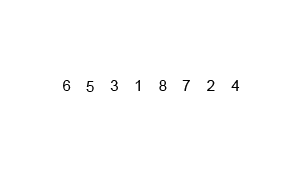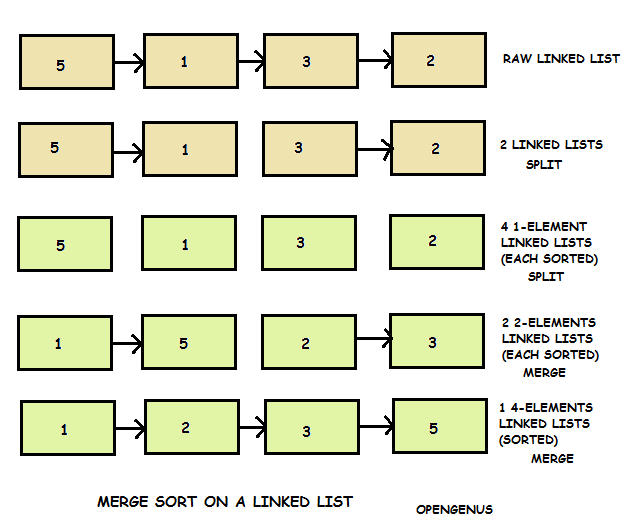×

Search anything:

# Merge Sort

#### Sorting Algorithms merge sort AlgorithmsReading time: 20 minutes | Coding time: 10 minutes

The Merge Sort is an efficient comparison based sorting algorithm based on divide and conquer strategy. It has a usual performance of `Θ(N log N)`. Apart from the optimal speed, Merge Sort has found usage in parallel applications and seem to be the choice of sorting algorithm despite the tough competition posed by other optimal algorithms like Quick Sort. In fact, Merge sort does about 39% fewer comparisons than Quick Sort in the average case.

### Complexity

The time and space complexity of Merge Sort are:

• Worst case time complexity: `Θ(N log N)`
• Average case time complexity: `Θ(N log N)`
• Best case time complexity: `Θ(N log N)` (naive); `Θ(N)` (natural variant)
• Space complexity: `Θ(N)` (auxillary); `Θ(1)` (using linked lists)

Read ahead regarding the algorithm and make sense of the complexity depicted above. You can always ask us questions.

Merge Sort was invented by John von Neumann in 1945.### Algorithm

Follow these steps to sort any data using Merge Sort:

Step 1: Divide the unsorted list into n sublists; each sublist has one element (which is sorted as it has one element)

Step 2: Merge two lists at a time. While merging compare elements for the two sublists and prepare a new sorted list. This takes linear time.

Step 3: Do step 2 for all sub-list pairs

Step 4: Repeat step 2 to 3 for the new list of sub-lists until we have only one list

### Example

``````
Consider the following list of integers:

(2 4 1 6) (we want to sort it in ascending order)

Split the list into N sublists (step 1)
New list: (2) (4) (1) (6)

Step 2 and 3: Merge list in pairs of two.
Merge (2) (4) : (2 4)
Merge (1) (6) : (1 6)
New list: (2 4) (1 6)

Step 2: Merge the two lists (2 4) (1 6)
Compare 2 and 1: As 1 is smaller, it is inserted in the new list (1)
Compare 2 and 6: As 2 is smaller, it is inserted in the new list (1 2)
Compare 4 and 6: As 4 is smaller, it is inserted in the new list (1 2 4)
Only one element 6 is left, so insert it in the new list (1 2 4 6)

Entire list is sorted
``````

### Visual RunExample of Merge Sort on a Linked List:### Pseudocode

``````
Merge_Sort(array[], left_end,  right_end)
If right_end > left_end
1. Find the middle point to divide the array into two halves:
middle = (left_end + right_end)/2
2. Call Merge_Sort for first half:
Call Merge_Sort(array, left_end, middle)
3. Call Merge_Sort for second half:
Call Merge_Sort(array, middle + 1, right_end)
4. Merge the two halves sorted in step 2 and 3:
Call Merge(array, left_end, middle, right_end)

// Procedure to merge two sorted array in sorted order
Merge(array[], left_end, middle, right_end)
left_i = 1, right_i = middle
while(left_i < left_end AND right_i < right_end)
if(element at left_i > element at right_i)
left_i ++
else if(element at left_i < element at right_i)
left_i ++ and right_i ++

``````

### Implementations

Implementation of Merge Sort algorithm in 5 languages that includes `C`, `C++`, `Java`, `Python` and `C#`.

• C
• C++
• Java
• Python
• C#

### C

``````
/*
* Part of Cosmos by OpenGenus Foundation
*/
#include <stdio.h>
#define max 10
int a = { 10, 14, 19, 26, 27, 31, 33, 35, 42, 44, 0 };
int b;
void merging(int low, int mid, int high) {
int l1, l2, i;
for(l1 = low, l2 = mid + 1, i = low; l1 <= mid && l2 <= high; i++) {
if(a[l1] <= a[l2])
b[i] = a[l1++];
else
b[i] = a[l2++];
}
while(l1 <= mid)
b[i++] = a[l1++];
while(l2 <= high)
b[i++] = a[l2++];
for(i = low; i <= high; i++)
a[i] = b[i];
}
void sort(int low, int high) {
int mid;
if(low < high) {
mid = (low + high) / 2;
sort(low, mid);
sort(mid+1, high);
merging(low, mid, high);
} else {
return;
}
}
int main() {
int i;
printf("List before sorting\n");
for(i = 0; i <= max; i++)
printf("%d ", a[i]);
sort(0, max);
printf("\nList after sorting\n");
for(i = 0; i <= max; i++)
printf("%d ", a[i]);
}
``````

### C++

``````
/*
Part of Cosmos by OpenGenus Foundation
merge sort synopsis
namespace merge_sort_impl {
template<typename _Random_Acccess_Iter>
_Random_Acccess_Iter
}
template<typename _Random_Acccess_Iter, typename _Compare>
void mergeSort(_Random_Acccess_Iter begin, _Random_Acccess_Iter end, _Compare comp);
template<typename _Random_Acccess_Iter>
void mergeSort(_Random_Acccess_Iter begin, _Random_Acccess_Iter end);
*/
#include <list>
#include <iterator>
#include <cstddef>
namespace merge_sort_impl {
template<typename _Random_Acccess_Iter>
_Random_Acccess_Iter
{
return it;
}
template<typename _Random_Acccess_Iter, typename _Compare>
void merge(_Random_Acccess_Iter begin,
_Random_Acccess_Iter end,
_Compare comp)
{
using value_type = typename std::iterator_traits<_Random_Acccess_Iter>::value_type;
auto end1 = begin;
auto begin1 = begin,
begin2 = end1,
end2 = end;
std::list<value_type> tmp{};
while (begin1 < end1 &&  begin2 < end2)
tmp.push_back(comp(*begin1, *begin2) ? *begin1++ : *begin2++);
while (begin1 < end1)
*--begin2 = *--end1;
while (!tmp.empty())
{
*--begin2 = tmp.back();
tmp.pop_back();
}
}
}
template<typename _Random_Acccess_Iter, typename _Compare>
void
mergeSort(_Random_Acccess_Iter begin, _Random_Acccess_Iter end, _Compare comp)
{
size_t dist = std::distance(begin, end);
if (dist > 1)
{
auto mid = begin;
mergeSort(begin, mid, comp);
mergeSort(mid, end, comp);
merge_sort_impl::merge(begin, end, comp);
}
}
template<typename _Random_Acccess_Iter>
void
mergeSort(_Random_Acccess_Iter begin, _Random_Acccess_Iter end)
{
using value_type = typename std::iterator_traits<_Random_Acccess_Iter>::value_type;
mergeSort(begin, end, std::less<value_type>());
}
``````

### Java

``````
/* Java program for Merge Sort */
// Part of Cosmos by OpenGenus Foundation
class MergeSort {
// Merges two subarrays of arr[].
// First subarray is arr[l..m]
// Second subarray is arr[m+1..r]
private void merge(int arr[], int l, int m, int r) {
// Find sizes of two subarrays to be merged
int n1 = m - l + 1;
int n2 = r - m;
/* Create temp arrays */
int L[] = new int [n1];
int R[] = new int [n2];
/*Copy data to temp arrays*/
System.arraycopy(arr, l + 0, L, 0, n1);
for (int j=0; j<n2; ++j)
R[j] = arr[m + 1+ j];
/* Merge the temp arrays */
// Initial indexes of first and second subarrays
int i = 0, j = 0;
// Initial index of merged subarry array
int k = l;
while (i < n1 && j < n2) {
if (L[i] <= R[j]) {
arr[k] = L[i];
i++;
}
else {
arr[k] = R[j];
j++;
}
k++;
}
/* Copy remaining elements of L[] if any */
while (i < n1) {
arr[k] = L[i];
i++;
k++;
}
/* Copy remaining elements of R[] if any */
while (j < n2) {
arr[k] = R[j];
j++;
k++;
}
}
// Main function that sorts arr[l..r] using
// merge()
private void sort(int arr[], int l, int r) {
if (l < r) {
// Find the middle point
int m = (l+r)/2;
// Sort first and second halves
sort(arr, l, m);
sort(arr , m+1, r);
// Merge the sorted halves
merge(arr, l, m, r);
}
}
/* A utility function to print array of size n */
private static void printArray(int arr[]) {
int n = arr.length;
for (int anArr : arr) System.out.print(anArr + " ");
System.out.println();
}
// Driver method
public static void main(String args[]) {
int arr[] = {12, 11, 13, 5, 6, 7};
System.out.println("Given Array");
printArray(arr);
MergeSort ob = new MergeSort();
ob.sort(arr, 0, arr.length-1);
System.out.println("\nSorted array");
printArray(arr);
}
}
``````

### Python

``````
# Part of Cosmos by OpenGenus Foundation
# Split array in half, sort the 2 halves and merge them.
# When merging we pick the smallest element in the array.
# The "edge" case is when one of the arrays has no elements in it
# To avoid checking and breaking the look, finding which array has elements and then
# Starting another loop, we simply alias the arrays. This abstracts the issue without
# Creating a mess of code. When we alias, we decide with Xor which array to pick,
# Since we cover 0 ^ 0 case in the beginning of the loop,
# while bool(a) or bool(b) makes 0^0 is impossible to happen, Xor has 3 cases, 1 ^ 0, 0 ^ 1, 1^1 to evaluate.
# Since the 2 first cases are the edge we go inside the loop and alias the array that has elements in it.
# If it's 1^1 we simply pick the array with the smallest element.
from random import randint
def merge_sort(array):
if len(array) == 1:
return array
left = merge_sort(array[:(len(array)//2)])
right = merge_sort(array[(len(array)//2):])
out = []
while bool(left) or bool(right):
if bool(left) ^ bool(right):
alias = left if left else right
else:
alias = left if left < right else right
out.append(alias)
alias.remove(alias)
return out
randomized: list = [randint(0, 1000) for x in range(10000)]
clone = [x for x in randomized]
assert sorted(clone) == merge_sort(randomized)
``````

### Go

``````
package main
import (
"fmt"
)
func main() {
A := []int{3, 5, 1, 6, 1, 7, 2, 4, 5}
fmt.Println(sort(A))
}
// top-down approach
func sort(A []int) []int {
if len(A) <= 1 {
return A
}
left, right := split(A)
left = sort(left)
right = sort(right)
return merge(left, right)
}
// split array into two
func split(A []int) ([]int, []int) {
return A[0:len(A) / 2], A[len(A) / 2:]
}
// assumes that A and B are sorted
func merge(A, B []int) []int {
arr := make([]int, len(A) + len(B))
// index j for A, k for B
j, k := 0, 0
for i := 0; i < len(arr); i++ {
// fix for index out of range without using sentinel
if j >= len(A) {
arr[i] = B[k]
k++
continue
} else if k >= len(B) {
arr[i] = A[j]
j++
continue
}
// default loop condition
if A[j] > B[k] {
arr[i] = B[k]
k++
} else {
arr[i] = A[j]
j++
}
}
return arr
}
``````

### Applications

• Merge Sort is useful for sorting linked lists in O(n Log n) time

• Merge sort can be implemented without extra space for linked lists

• Merge sort is used for counting inversions in a list

• Merge sort is used in external sorting

### Questions

#### If we have unlimited computers for parallel sorting, what will be the time complexity in a clever use of Merge Sort?

O( (log N)^3 )
O( N log N )
O( 1 )
O( N )#### Alexa Ryder

Hi, I am creating the perfect textual information customized for learning. Message me for anything.

Improved & Reviewed by: